Updating search results...

# 6 Results

View
Selected filters:
• standard-form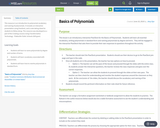Conditional Remix & Share Permitted
CC BY-NC-SA
Rating
0.0 stars

This resource is an introduction to polynomial vocabulary and naming of polynomials. It includes an interactive presentation using Pear Deck and a note taking sheet for students.

This resource was developed as a part of the Creating Lessons Using Transformative Technology - Platteville Public Schools OER grant.

Subject:
Mathematics
Material Type:
Lesson Plan
08/02/2019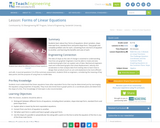Educational Use
Rating
0.0 stars

Students learn about four forms of equations: direct variation, slope-intercept form, standard form and point-slope form. They graph and complete problem sets for each, converting from one form of equation to another, and learning the benefits and uses of each.

Subject:
Algebra
Career and Technical Education
Mathematics
Technology and Engineering
Material Type:
Lesson Plan
Provider:
TeachEngineering
Provider Set:
TeachEngineering
Author:
Aubrey McKelvey
TeachEngineering.org
VU Bioengineering RET Program, School of Engineering,
09/18/2014Rating
0.0 stars

This task shows three equivalent expressions and requires that students understand the structure and purpose of each one. This is a critical aspect of Seeing Structure in Expressions.Â
The primary purpose of this task is to assess students' knowledge of certain aspects of the mathematics described in the High School domain A-SSE: Seeing Structure in Expressions. Specifically, standard A-SSE.3 reads A-SSE.3: Choose and produce an equivalent form of an expression to reveal and explain properties of the quantity represented by the expression. a. Factor a quadratic expression to reveal the zeros of the function it defines. b. Complete the square in a quadratic expression to reveal the maximum or minimum value of the function it defines.
The Standards avoid the term "simplify" as there isn't always an obvious simplest form. The emphasis instead is on, "purposeful transformation of expressions into equivalent forms that are suitable for the purpose at hand." This task shows three equivalent expressions and requires that students understand the structure and purpose of each one. This is a critical aspect of Seeing Structure in Expressions.Â
â€¢ Prompts students to analyze three equivalent quadratic expressions â€¢ Allows students to focus on the structure of the expressions without focusing on the procedural skill of factoring or expanding the expressions â€¢ Gives a real-world context for students to interpret the properties of quadratic expressions â€¢ Requires students to look for and make use of structure (MP.7)
In the classroom: â€¢ Offers students and teachers an opportunity to see an assessment-type task with two response types â€¢ Allows teachers to target specific student misunderstandings for reteaching â€¢ With follow-up questions, teachers can prompt students to share their thinking about the concepts in this task

Subject:
Mathematics
Material Type:
Alternate Assessment
Formative Assessment
Provider:
Achieve the Core
06/04/2014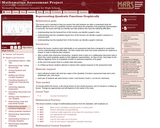Only Sharing Permitted
CC BY-NC-ND
Rating
0.0 stars

Mathematical goals
This lesson unit is intended to help you assess how well students are able to understand what the different algebraic forms of a quadratic function reveal about the properties of its graphical representation. In particular, the lesson will help you identify and help students who have the following difficulties:
Understanding how the factored form of the function can identify a graph's roots.Understanding how the completed square form of the function can identify a graph's maximum or minimum point.Understanding how the standard form of the function can identify a graph's intercept.

Before the lesson, students work individually on an assessment task that is designed to reveal their current understandings and difficulties. You then review their work and create questions for students to answer in order to improve their solutions.After a whole-class interactive introduction, students work in pairs on a collaborative discussion task in which they match quadratic graphs to their algebraic representation. As they do this, they begin to link different algebraic forms of a quadratic function to particular properties of its graph.At the end of the lesson there is a whole-class discussion.In a follow-up lesson students attempt to improve their original response to the assessment task.
Materials required
Each individual student will need two copies of the Quadratic Functions assessment task and a mini-whiteboard, pen, and eraser.Each pair of students will need Domino Cards 1 and Domino Cards 2, cut into ten Ã¢â‚¬Ëœdominoes'.
Time needed
15 minutes before the lesson, a 95-minute lesson (or two shorter lessons), and 10 minutes in a follow-up lesson. Timings are approximate and will depend on the needs of the class.

Subject:
Mathematics
Material Type:
Alternate Assessment
Assessment Item
Formative Assessment
Game
Lesson Plan
Self Assessment
Provider:
Shell Center for Mathematical Education
10/28/2015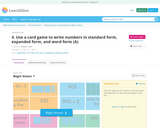Rating
0.0 stars

Lesson objective: Apply knowledge of ways to name numbers.
This lesson provides an opportunity for students to apply their knowledge and understanding of naming numbers to a mathematical situation. Students are asked to help the teacher complete her set of game cards by coming up with equivalent names for numbers.
Key Concept students will use:
Representing a whole number in different ways (including base ten numerals, number-names, and expanded form) does not change its value.
Skills students will use:
Understand that the three digits of a three-digit number represent the amount of hundreds, tens, and ones (Grade 2, Unit 6)Write three-digit numbers in expanded formWrite three-digit numbers in word formWrite three-digit numbers in standard form
Students engage in Mathematical Practice 7 (Look for and make use of structure) as they look for patterns in the way we name numbers to name numbers they have not seen before.

Subject:
Mathematics
Material Type:
Lesson Plan
Provider:
Learn Zillion
03/20/2018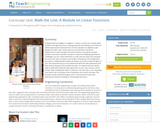Educational Use
Rating
0.0 stars

Subject:
Algebra
Career and Technical Education
Life Science
Mathematics
Technology and Engineering
Material Type:
Unit of Study
Provider:
TeachEngineering
Provider Set:
TeachEngineering
Author:
Aubrey Mckelvey
TeachEngineering.org
VU Bioengineering RET Program,